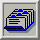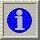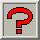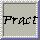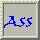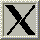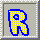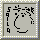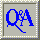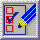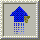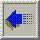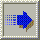##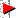3.10. Octree Quantisation

From Glassner (1990) :

The principle of the octree quantisation method is as follows. The image is read in sequentially. The first K different colours are used as initial entries to the colour table. If another colour is added, which means that the already processed part of the image has K + 1 different colours, some very near neighbours are merged into one and substituted by their mean. This step is repeated for every additional colour, so that at any moment no more than K representatives are left. This property, of course, remains true after the image is completely processed.

The algorithm is done in three phases:

• evaluation of the representatives
• filling the colour table
• mapping the original colours onto the representatives.

These will now be looked at in greater detail.

### 3.10.1. Evaluation of the Representatives

The octree is constructed only in those parts that are necessary for the image we want. At the beginning, the octree is empty. Every colour that occurs in the image is now inserted by generating a leaf in depth eight; thereby, the colour is represented exactly.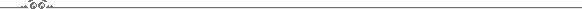```  InsertTree(Tree:Octree,RGB:Colour);
inserts the colour RGB into the subtree Tree

begin InsertTree
if Tree = nil then
NewAndInit (Tree); produces and inits a new octree node.
if Leaf then              we have reached the eighth level.
begin
inc(Tree^.ColourCount);   update the number of represented pixels
end
else InsertTree (Next[Branch(RGB)],RGB);
end;
```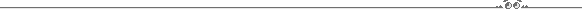In this way, an incomplete octree is created, in which many branches are missing. Actually, this octree does not have to be filled with all the colours because every time the number of colours reaches K + 1, similar colours can be merged into one, so that there are never more than K colours left. We will call this action a reduction of the octree.```  ReduceTree combines the successors of an intermediate node to
one leaf node
GetReducible(Tree);               finds a reducible node
Sum <- (0,0,0);
Children <- 0;
for i:integer <- 0, 7 do
if Next[i] <> nil then  there is an ith successor
begin
Children <- Children + 1;
end
Tree^.Leaf <- true;           cut the tree at this level
Tree^.RGB <- Sum;             the node represents the sum of all colour values
Size <- Size - Children + 1;  of children

```Every time the number of leaves (that is, the number of representatives found up to the moment) exceeds K, the octree is reduced. The reduction begins at the bottom of the octree by always substituting some leaves by their predecessor.

Reducing the octree, the following criteria are relevant:

• From all reducible nodes, those that have the largest depths within the octree should be chosen first, for they represent colours that lie closest together.

• If there is more than one node in the largest depth, additional criteria could be used for an optimal selection. For example, reduce the node that represents the fewest pixels up to now. In this way the error sum will be kept small. Reduce the node that represents the most pixels up to now. In this case large areas will be uniformly filled in a slightly wrong colour, and detailed shadings (like anti-aliasing) will remain.

To construct the octree, the whole image has to be read once and all colours of the image have to be inserted into the octree.

### 3.10.2. Filling the Colour Table

At the end, the K leaves of the octree contain the colours for the colour table (the mean value of all represented colours = RGB/ColourCount). They can be written into the colour table by recursively examining the octree. During this recursive tree traversal in every leaf node of the octree also its own colour index is stored.

### 3.10.3. Mapping the Original Colours onto the Representatives

The mapping of the original colours onto their representatives can now be managed easily with the octree, too. Trying to find any original colour in the reduced octree will end at a leaf in some depth. This node contains a colour very similar to the one in search, and is therefore its representative. Since the index of the colour table is stored there too, no further search has to be carried out.

If the original image used less than K colours, no reduction will have taken place, and the found colour table entry will contain exactly the correct colour. Otherwise, only the path to the leaf in depth eight was shortened by the reduction, so that the colour will be displayed less exactly by the means of all the colours that had their paths over this node. Since the octree contains only K leaves, all original colours are mapped onto valid colour table entries. For this, the image has to be read a second time.

The visual result using this octree quantisation is of similar quality as the result using the median cut method.```  Quant (Tree:Octree,Original_Colour:Colour):index;
for the original colour its representative
is searched for in the octree, and the index of its
colour table entry is returned

begin Quant
if Leaf then
return Tree^.ColourIndex stored colour index in the tree
else
return Quant(Tree^.Next[Branch(Original_Colour)],Original_Colour)
end;

```### 3.10.4. Improvements

A significant portion of the time is spent with the search for an optimal reducible node every time a reduction of the octree has to take place. These nodes can be collected easily in an appropriate structure during the construction of the tree. They have to be sorted by depth to ensure quick access. An appropriate structure for this purpose has proved to be eight linear lists (one for every depth level) containing all reducible nodes. All nodes of one depth level are elements of the same list. The node with the largest depth can then be found quickly for reduction.

An intermediate node is inserted into its list of reducible nodes after its creation during NewAndInit.

At any given moment one level of the octree will be the depth in which the reductions take place. This depth is the level of the deepest intermediate nodes. At the beginning, this is level seven, and it moves toward the root during octree construction. This "reduction" level states what the minimal distance between two representatives will already have to be. This minimal distance can never again decrease even by adding more colours to the octree. Therefore, nothing beneath this reduction level + 1 will ever again be relevant so that the insertion of colours can also stop at that depth. The depth of the octree is not constant, but decreases with lifetime.

### 3.10.5. Memory and Computational Expense

Let N be the number of pixels of the original image. If the image is run-length encoded, N can also be the number of runs of the image. The algorithm has to be modified slightly by using runs instead of pixels in the octree.

Let K be the number of representatives, that is, the size of the colour table. Let D be the number of different colours in the original image. In general the following equations hold:```
N > D > K and N >> K.

```An upper bound for the memory used by the octree is 2.K - 1 nodes, because there are K leaves and at the most (in the case of a bintree) K - 1 intermediate nodes. The algorithm needs very little memory! It is also independent of N and D, that is, of the image. Only the colour table size is relevant.

Upper bounds for the number of steps for the insertions, for the generation of the colour table and for the quantisation are:```
Insertion:N.MaxDepth.

```N insertions take place, each of them not deeper than MaxDepth. MaxDepth itself is a constant (&lt = 8).```
Colour table generation:2.K.

```